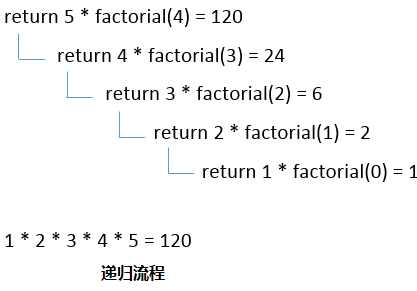# C++函数递归

```recursionfunction(){
recursionfunction(); //calling self function
}
```

## C++递归示例

```#include<iostream>
using namespace std;
int main()
{
int factorial(int);
int fact,value;
cout<<"Enter any number: ";
cin>>value;
fact=factorial(value);
cout<<"Factorial of a number is: "<<fact<<endl;
return 0;
}

int factorial(int n)
{
if(n<0)
return(-1); /*Wrong value*/
if(n==0)
return(1);  /*Terminating condition*/
else
{
return(n*factorial(n-1));
}
}
```

```Enter any number: 5
Factorial of a number is: 120```